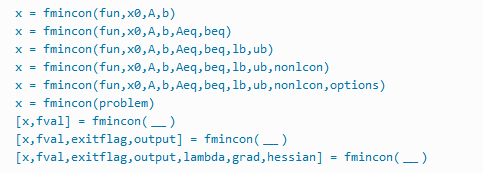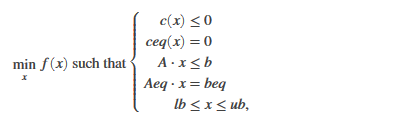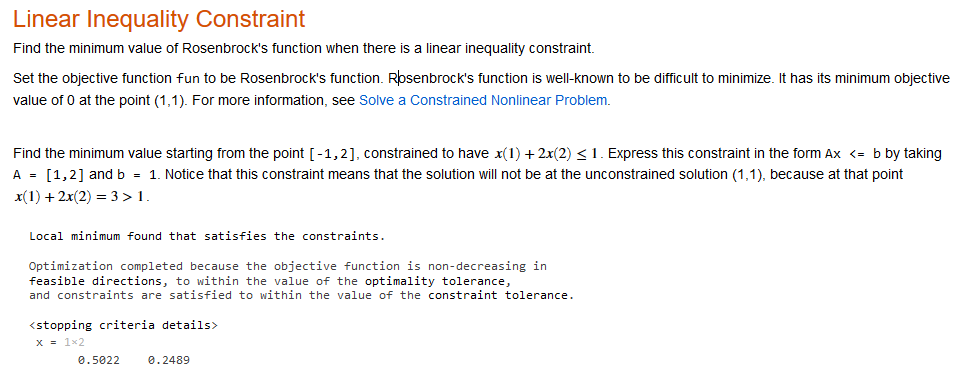Showing posts with label fmincon matlab example. Show all posts
Showing posts with label fmincon matlab example. Show all posts

## fmincon Matlab Function With fmincon Example

fmincon function in MATLAB is used to find the minimum of any given nonlinear multivariable function.

MATLAB Syntax : -MATLAB Syntax

## Description For fmincon MATLAB

Nonlinear programming solver.

Let see the a problem here and find the minimum value for this problem.fmincon MATLAB

Well b and beq are vectors, A and Aeq are matrices in this given equation on the other hand c(x) and ceq(x) are the functions in equation that return vectors.

well as you can see in the equation f(x) is a function which returns a scalar and remember all the three function f(x),ceq(x), and c(x) are non linear.

lb,x and ub can be passed as vectors or matrices in the fmincon MATLAB.

•  x = fmincon(fun,xθ,A,b) function is started with the xθ (x theta) and try to find the minimizer x for the given function and the expression for this  A*x ≤ b. xθ can be a scalar, vector, or matrix.
•  x = fmincon(fun,x0,A,b,Aeq,beq) minimize this function to linear equalities Aeq*x = beq and A*x ≤ b.If there is no inequalities exist, then set A = [] and b = [].
• x = fmincon(fun,x0,A,b,Aeq,beq,lb,ub) helps to define the lower and the upper case on the design variables in x, that's why the solution always exixt in between lb ≤ x ≤ ub.
x = fmincon(fun,x0,A,b,Aeq,beq,lb,ub,nonlcon) will helps to minimization to the nonlinear inequalities c(x) or the equalities ceq(x) defined in nonlcon.

x = fmincon(fun,x0,A,b,Aeq,beq,lb,ub,nonlcon,options) function helps to minimizes with the optimization options which is specified in options.

x = fmincon(problem) helps to finds the minimum for the given problem, and a structure described in problem.

[x,fval,exitflag,output] = fmincon(___) will helps to return a value exitflag that describes the exit condition of fmincon function MATLAB.

# Example for fmincon function MATLAB

Find the minimum value of Rosenbrock's function if there is a linear inequality constraint.Example for fmincon function MATLAB